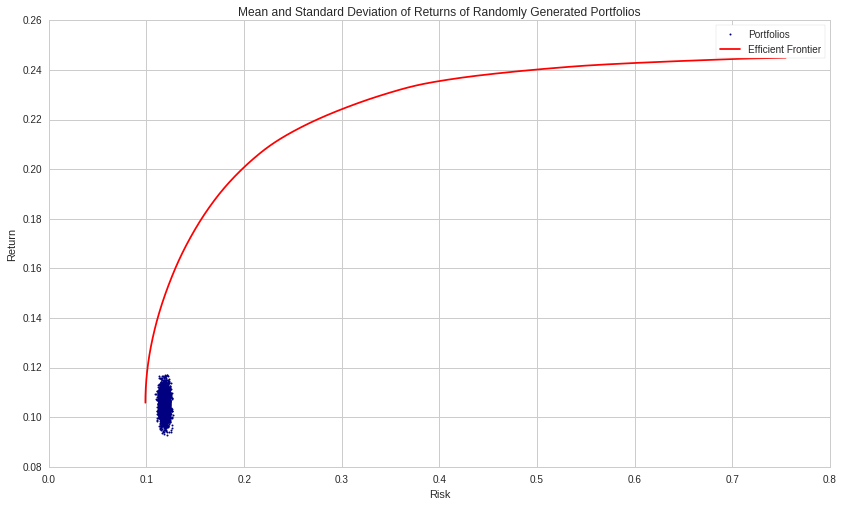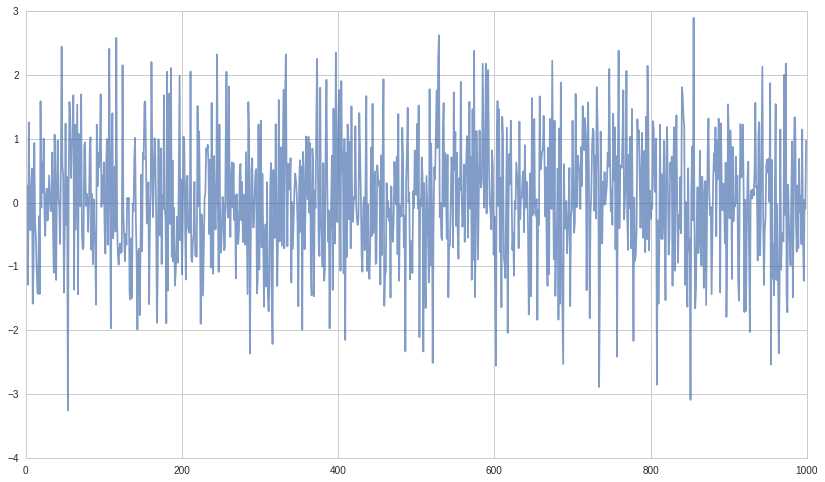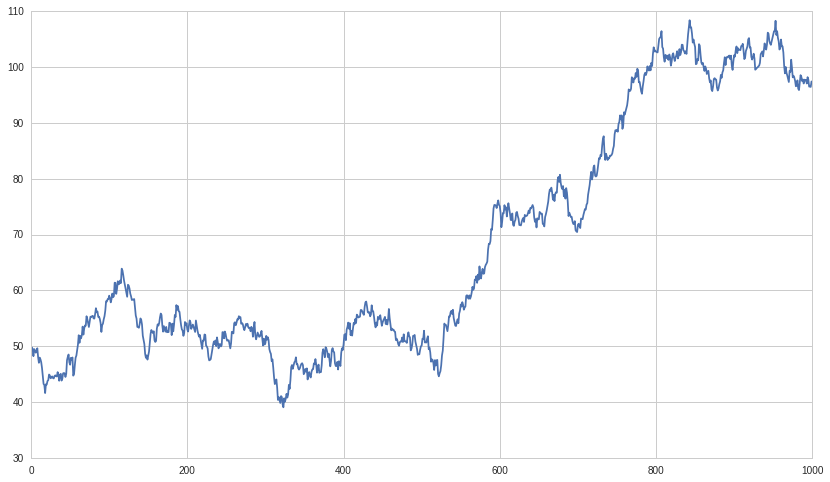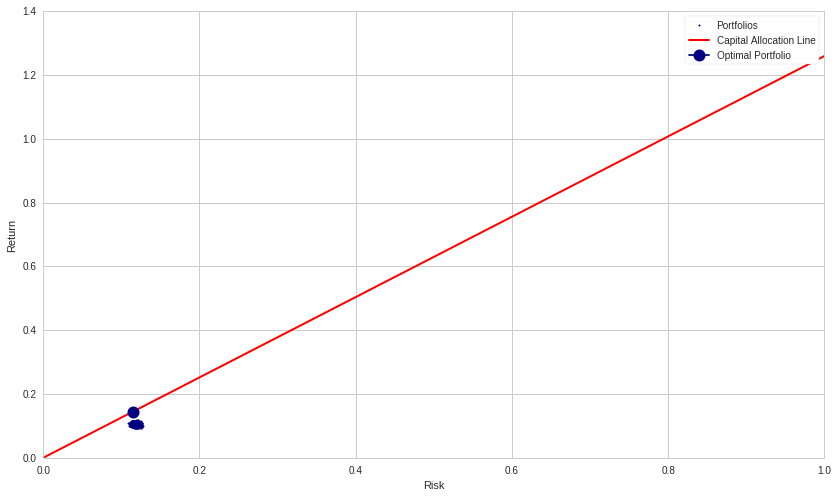# The CAPM Revisited II¶

Following the previous notebook, it was time to elaborate on what else was implied by those charts and findings.

If a Markowitz bullet illustrates the concept behind a portfolio's efficient frontier, then dealing only with the residuals was not enough. You needed a trend to show it had something. Otherwise, what you got was as presented in the previous notebook: not much. Just a statistical blob of portfolios centered around the zero-return line. Or, you could view it as portfolios trying very hard to reduce variance and volatility to such an extent that they succeeded in doing so.

Evidently, that was under the condition that prices series were the result of normally distributed random-like price series where no trends were even considered. Nonetheless, by commenting out the random seed: # np.random.seed(123) in the code module below, one could have ran as many different scenarios as they wished. And would have found that most ended about the same or quite close to each other. In fact, stating that the optimizer could not find anything in the residuals.

Using an optimizer on normally distributed price functions, as the one used in that notebook, did squash performance as you increased the number of stocks in the portfolio. And that should have been expected.

The Markowitz bullet simply shrank and migrated toward a zero-density expectancy as the number of stocks in the portfolio was increased.

That is not good news. Regardless, it is what it should have done, and it did.

The task of any portfolio manager, or a stock trading program, is to determine the allocation to any one stock for some duration. It should be done based on merit and expectancy. If you do not have a positive expectancy, why place a bet or invest in that stock in the first place?

The objective, whatever the method of play, is to make a profit: $\;q \cdot \Delta p > 0$. That you are long or short ($\,q\,$ or $\,-q\,$) does not change the profit definition.

A portfolio of stocks to show a profit needs to sum the outcome of all its trades and positions: $\sum_i (q_i \cdot \Delta^i p_i) > 0$. If this sum is not greater than zero, whatever the trading method, the strategy was most certainly not profitable. There is not much to add to this: in the end (over the long run) whatever our programs do, either a portfolio of stocks is profitable OR it is not!

We do simulations to find out how our trading strategies would behave IF. You are not looking for precise answers. It is more like how it will behave? Every scenario is a "what if" this or that happened and then what? Doing simulations is a lot less expensive than trying it out live. But still, our simulation environments should aspire to be a representation of the real world. So, one question should be: how realistic is this?

The previous notebook established that if you did not have some underlying trend in a diversified group of stocks, you might have had nothing return wise, or close to it. You need something to break the random component of the stochastic price function, otherwise, you are left with a quasi-Gaussian distribution. If you cannot predict future prices over the very short-term then the end results could be considered as close to the equivalent.

Over the short-term, most of the long-term trends are drown in all the noise. And over the short-term, the ability to extract the signal from the noise is rather limited. Most often, the signal to noise ratio is so low, it is difficult to even identify it.

A normalized randomly generated distribution of returns has an expectancy of zero. No matter the length of the sequence, the expectancy remains zero. That you throw in an optimizer in an attempt to find something in it does not matter, there is nothing to be had in the first place.

The closer the distribution of trades approaches 50/50, the closer it has the characteristics of a quasi-normal distribution. It will be something that will have a tendency to show about half of the returns going up and the other half going down.

You do not need to add much upside for the optimizer to show an advantage since a slight trend could be partially picked up by the optimizer and be taken advantage of. It would be like putting back $\,\mu dt\,$ into the stock's stochastic equation: $\;p(t) = \mu dt + \sigma dW$. Let's do that. The two main numbers of interest in this notebook are drift and alpha.

In :
# Import libraries
import numpy as np
import pandas as pd
import statsmodels.api as sm
from statsmodels import regression
import matplotlib.pyplot as plt

from scipy import optimize
import cvxopt as opt
from cvxopt import blas, solvers
import random


Take the scenario from the previous notebook using 100 securities to which is added a long-term linear drift. The optimizer will not be able to take advantage of it all, but still, it will be sufficient to move the average portfolio's center of mass up (expected average return) as depicted in the chart below.

In :
# np.random.seed(123)

# Turn off progress printing
solvers.options['show_progress'] = False

alpha = 0.000   # 0.001

# Number of assets
n_assets = 100

# Number of observations
n_obs =1000

# Generating random returns for the securities
return_vec = np.random.randn(n_assets, n_obs)
drift = np.zeros((n_assets, n_obs))
drift += 0.002 + alpha
# add a linear drift (µdt)
drift = np.cumsum(drift, axis=0)
return_vec = return_vec + drift

def rand_weights(n):
'''
Produces n random weights that sum to 1
'''
k = np.random.rand(n)
return k / sum(k)

def random_portfolio(returns):
'''
Returns the mean and standard deviation of returns for a random portfolio
'''

p = np.asmatrix(np.mean(returns, axis=1))
w = np.asmatrix(rand_weights(returns.shape))
C = np.asmatrix(np.cov(returns))

mu = w * p.T
sigma = np.sqrt(w * C * w.T)

# This recursion reduces outliers to keep plots pretty
if sigma > 2.0:
return random_portfolio(returns)
return mu, sigma

def optimal_portfolios(returns):
n = len(returns)
returns = np.asmatrix(returns)

N = 1000

# Creating a list of returns to optimize the risk for
mus = [100**(5.0 * t/N - 1.0) for t in range(N)]

# Convert to cvxopt matrices
S = opt.matrix(np.cov(returns))
pbar = opt.matrix(np.mean(returns, axis=1))

# Create constraint matrices
G = -opt.matrix(np.eye(n))   # negative n x n identity matrix
h = opt.matrix(0.0, (n ,1))
A = opt.matrix(1.0, (1, n))
b = opt.matrix(1.0)

# Calculate efficient frontier weights using quadratic programming
portfolios = [solvers.qp(mu*S, -pbar, G, h, A, b)['x']
for mu in mus]

## Calculate the risk and returns of the frontier
returns = [blas.dot(pbar, x) for x in portfolios]
risks = [np.sqrt(blas.dot(x, S*x)) for x in portfolios]

return returns, risks

n_portfolios = 5000

means, stds = np.column_stack([random_portfolio(return_vec) for x in range(n_portfolios)])

returns, risks = optimal_portfolios(return_vec)

plt.plot(stds, means, 'o', markersize=2, color='navy')
plt.xlabel('Risk')
plt.ylabel('Return')
plt.title('Mean and Standard Deviation of Returns of Randomly Generated Portfolios');

plt.plot(risks, returns, '-', markersize=3, color='red');
plt.legend(['Portfolios', 'Efficient Frontier']);

# Markowitz Bullet:  100 StocksThe code does not say what is the origin of the drift, only that it is there, and linear. 5,000 portfolios were generated, yet, they are all clustered together and spread over a small vertical slice or risk.

The cluster of portfolios could be even more concentrated simply by adding more stocks to the mix since the size of the cluster is related to the bet size. Smaller average bet size, smaller and more compact clusters.

Only a statistical part of the drift was detected by the optimizer and taken advantage of. Moving the cluster of portfolios higher the return space for about the same level of risk. And it did not change the signature of the return vector by much as the next chart shows.

In :
# Plot a single return vector (normally distributed).
# Pick a number between 0 and (n_assets -1).
pick_one = False
if pick_one:
picked_ret_vec = 42
else:
# or get a random selection from set
picked_ret_vec = random.randrange(0,n_assets -1)
plt.plot(return_vec[picked_ret_vec], alpha = 0.7);
print 'Randomly Selected Stock Number: ', picked_ret_vec

Randomly Selected Stock Number:  16Re-run the above code snippet to see another stock's quasi-random return-stream.

We can see the impact of the added trend in its corresponding price chart (next code snippet).

In :
#Plot selected return stream as if an index, since only the percentages are of interest.
plt.plot(np.cumsum(return_vec[picked_ret_vec])+50);
print 'Randomly Selected Stock Number: ', picked_ret_vec

Randomly Selected Stock Number:  16## The Drift¶

The funny thing is that the drift comes with the game itself, or at least, some of it as built-in. And the optimizer can catch some of it. If we exclude the drift from our trading procedures by demeaning price series and only trying to find "factors" in the "residuals", this becomes the same as having transformed our price series to quasi-Gaussian distribution. And as such, should produce the charts of the last notebook.

In fact, saying that there is no profit to be had if there is no drift. Therefore, excluding it from structural strategy design might not be the most promising. However.

What about if you bring in some alpha of your own? As in: $p(t) = (\mu + \alpha)dt + \sigma dW$. By adding some skills to the mix, you can raise the bar: drift += 0.002 + alpha. Just a little alpha above the drift can make quite a difference. Making add_drift = False will eliminate any consideration for the drift and alpha making the program behave exactly like in the previous notebook.

Another thing that should be considered. If the drift is there for the taking, just for participating in the game, and that any method of play can catch part of it, are you really exercising some skills or just going along for the ride?

In real life, you might not be able to separate the trend and the alpha generation of your trading strategy or strategies. However, one thing should be evident, and that is if you demean your price series to only consider detecting your alpha from the residuals, you will be missing out: $p(t) = \alpha dt + \sigma dW$ since your alpha will need to work much harder to compensate for what was thrown away. The optimizer could have made good use of that underlying trend, even if it can only catch part of it.

Should you not be able to generate some alpha, it will produce: $p(t) = \sigma dW$. Then, you would be right back in the previous notebook analyzing residuals which in aggregate would hover around the zero-return line.

## The Sharpe Ratio¶

The chart below tries to maximize the Sharpe ratio using the same program snippet as in the previous notebook.

In :
# first get a risk-free rate of return proxy
start_date = '2014-01-01'
end_date = '2018-12-31'

R_F = get_pricing('BIL', fields='price', start_date=start_date, end_date=end_date).pct_change()[1:]

def maximize_sharpe_ratio(return_vec, risk_free_rate):
"""
Finds the CAPM optimal portfolio from the efficient frontier
by optimizing the Sharpe ratio.
"""

def find_sharpe(weights):

means = [np.mean(asset) for asset in return_vec]

numerator = sum(weights[m]*means[m] for m in range(len(means))) - risk_free_rate

weight = np.array(weights)

denominator = np.sqrt(weights.T.dot(np.corrcoef(return_vec).dot(weights)))

return numerator/denominator

guess = np.ones(len(return_vec)) / len(return_vec)

def objective(weights):
return -find_sharpe(weights)

# Set up equality constrained
cons = {'type':'eq', 'fun': lambda x: np.sum(np.abs(x)) - 1}

# Set up bounds for individual weights
bnds = [(0, 1)] * len(return_vec)

results = optimize.minimize(objective, guess,
constraints=cons, bounds=bnds,
method='SLSQP', options={'disp': False})

return results

risk_free_rate = np.mean(R_F)

results = maximize_sharpe_ratio(return_vec, risk_free_rate)

# Applying the optimal weights to each assset to get build portfolio
optimal_mean = sum(results.x[i]*np.mean(return_vec[i]) for i in range(len(results.x)))

optimal_std = np.sqrt(results.x.T.dot(np.corrcoef(return_vec).dot(results.x)))

# Plot of all possible portfolios
plt.plot(stds, means, 'o', markersize=2, color='navy')
plt.ylabel('Return')
plt.xlabel('Risk')

# Line from the risk-free rate to the optimal portfolio
eqn_of_the_line = lambda x : ( (optimal_mean-risk_free_rate) / optimal_std ) * x + risk_free_rate

xrange = np.linspace(0., 1., num=11)

plt.plot(xrange, [eqn_of_the_line(x) for x in xrange], color='red', linestyle='-', linewidth=2)

# Our optimal portfolio
plt.plot([optimal_std], [optimal_mean], marker='o', markersize=12, color="navy")

plt.legend(['Portfolios', 'Capital Allocation Line', 'Optimal Portfolio']);Observe how the 5,000 portfolios are concentrated in a single spot. From randomly generated price series, they all clustered around their center of mass. There is still a Markowitz bullet in there and it is relatively close to the optimal portfolio. Also noteworthy, any one of the 5,000 portfolios could have been a good choice since their relative differences are quite small (in the order of rounding errors).

You could re-run the notebook programs as many times as you want. Each time generating 5,000 new portfolios with a new set of 100 randomly generated stock prices. The Markowitz bullet would still be close to the optimal portfolio. Indicating that it is not the price series that got it there, but the drift and the alpha: $\,\bar g = \mu + \alpha$.

You could redo all the above after adding some alpha. See code line: alpha = 0.000 in the second code module and make it alpha = 0.001 for instance. Then look at the difference it can make.
The problem one needs to consider is: where is the alpha? Not so much where is the drift since it is technically already built-in. We might not be able to separate the alpha from the drift: $\,\bar g = \mu + \alpha$. But, we can still get it all: $p(t) = \bar g dt + \sigma dW$.
$©$ October 2018. Guy R. Fleury